# Purpose

With MultIS, we present a bioinformatical approach to detect the multiple integration of viral vectors within the same clone. These integrations result in multiple integration sites (IS) that can be detected using sequencing methods and traced in time series data. Our method is based on the idea that read outs of IS within the same clone appear in similar relative frequencies to each other over different measurements, while IS from different clones will change their relative mutual frequency according to the relative clone sizes to which they belong. We calculate the correlation of these frequencies for all pairs of IS to quantify their similarity and subsequently use clustering algorithms to identify sets of IS with high internal correlation, suggesting the same clonal origin.

# MultIS with biological data

When using “MultIS” with biological data, the clonal data should be stored in a matrix data structure. To have easy access to the included plotting routines, simply assign this matrix the additional class “timeseries”. The class is the used to dispatch to the correct function.

For example, one can load a data set like this:

dat <- read.table(file = "example_readouts.csv",
sep = ",", header = TRUE, row.names = 1, check.names = FALSE)
dat <- as.matrix(dat)
class(dat) <- c(class(dat), "timeseries")


The rows in this matrix represent individual IS (or unique clonal identifiers, barcodes), while the columns represent the different measurements. Values in the matrix show the read count for the respective IS and measurement.

Within our package, measurement refers not only to different time points, but can also refer to measurements of different cell types.

Here, 13 consecutive measurements for ten IS of our example are shown:

0 60 120 180 240 300 360 420 480 540 600 660 720
1 115.33 304.72 654.23 382.76 319.27 518.79 146.84 247.09 461.34 488.91 156.06 306.41 132.95
2 99.22 362.06 730.92 341.82 210.45 351.11 231.11 210.25 394.02 520.90 131.29 352.96 155.78
3 117.08 352.31 837.02 415.17 393.18 345.97 175.57 184.10 430.09 456.06 109.34 415.21 150.27
4 144.68 375.95 671.71 417.10 334.81 474.34 210.42 210.31 426.96 607.31 169.89 261.81 141.64
5 136.15 389.61 497.48 387.32 317.22 332.69 128.00 144.66 536.87 338.37 122.65 252.73 178.14
6 106.31 521.91 465.26 370.76 250.67 401.90 190.75 202.49 430.62 563.36 142.02 285.21 118.11
7 103.34 561.13 605.81 251.26 314.98 361.70 165.23 187.68 377.06 650.66 176.16 390.83 162.99
8 147.81 645.80 354.44 218.60 1333.11 435.76 199.58 864.62 359.63 701.14 510.24 637.05 371.20
9 130.89 865.50 426.69 289.42 1456.88 515.69 239.24 902.85 565.68 714.20 328.25 477.05 442.06
10 136.15 558.03 500.25 222.31 1282.24 531.11 128.05 745.72 823.16 784.87 648.84 740.30 444.34

## Visualize a time course

Stacked area plots are implemented as a visual representation of the IS abundance over different measurements. Here, we show the relative readouts of the integration sites originating from the time course data.

plot(dat)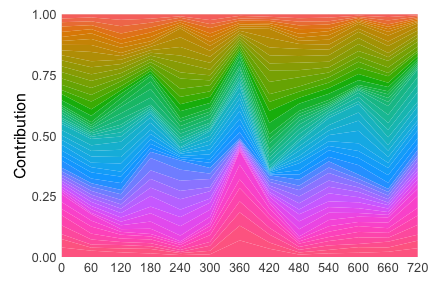## Apply filtering strategies

Optionally, the data can be filtered. This step is recommended to avoid the detection of spurious correlations. There are several filter functions:

• filter_at_tp_biggest_n selects for a number of most abundant IS at a specified measurement.
• filter_at_tp_min selects for IS that have at least a given number of reads in a certain measurement. The measurement is specified as a string and, if left empty, matches all IS that have that many reads in any measurement.
• filter_match selects for measurements that match a certain string.
• filter_nr_tp_min selects for IS that show in at least a given number of measurements.
• filter_zero_columns removes columns that have a sum of 0. This would eliminate measurements where no data is available. For example, after filtering for certain IS, some measurements might not hold any reads for these IS. filter_zero_rows removes rows that have a sum of 0. This would eliminate IS for which no data is available, e.g. after some measurements where removed and the IS did only show up in the removed measurements.

Here, we demonstrate how to apply a filter that selects for the 10 most abundant IS at the final timepoint.

filteredDat <- MultIS::filter_at_tp_biggest_n(dat, at = "720", n = 10L)
plot(filteredDat)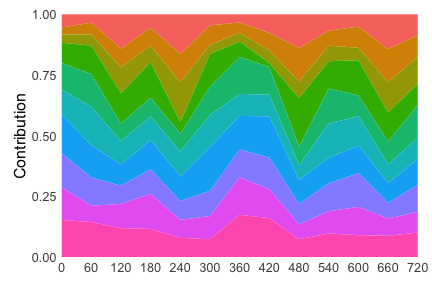## Calculate similarities between integration sites

Next, we can determine similarities between different IS. Here, we show the similarity based on $$R^2$$ illustrated by two integration sites (42, 43) originating from the same clone. They present a high $$R^2$$ similarity score. Two integration sites originating from different clones (43, 44) do not show this characteristic correlation:

r2 = round(summary(stats::lm(y ~ 0 + x, data = data.frame(
x = dat[is1, ], y = dat[is2, ])))$r.squared, 3) p1 <- MultIS::plot_rsquare(dat, is1, is2) + ggplot2::ggtitle(label = bquote(R^2 == .(r2))) + ggplot2::theme(plot.title = ggplot2::element_text(hjust = 0.5)) r2 = round(summary(stats::lm(y ~ 0 + x, data = data.frame( x = dat[is2, ], y = dat[is3, ])))$r.squared, 3)

p2 <- MultIS::plot_rsquare(dat, is2, is3) +
ggplot2::ggtitle(label = bquote(R^2 == .(r2))) +
ggplot2::theme(plot.title = ggplot2::element_text(hjust = 0.5))

gridExtra::grid.arrange(p1, p2, ncol = 2)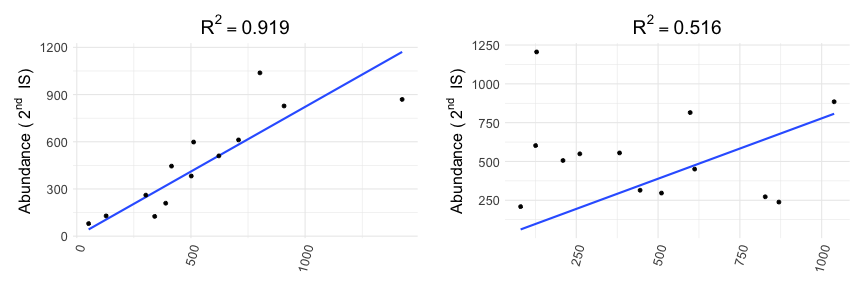Next, we calculate the similarity of all pairs of integration sites, which gives us the similarity matrix.

similarityMatrix <- MultIS::get_similarity_matrix(dat, parallel = FALSE)


The similarity matrix is conveniently visualized using a heatmap, where clusters of similar integration sites can already be seen:

plot(similarityMatrix)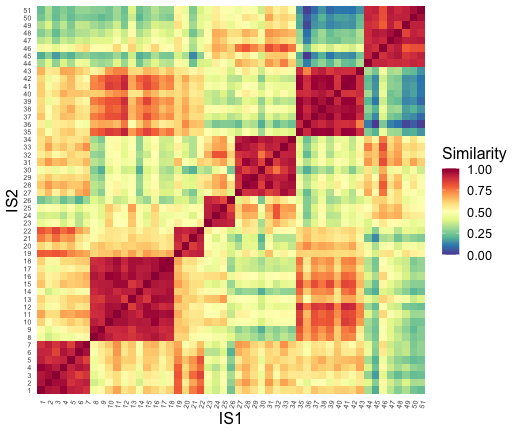## Clustering of similar integration sites

To represent the clusterings produced by the k-Medoids clustering algorithm, we visualize them for a defined number of clusters ($$k = 2$$ and $$k = 4$$):

clusterObjC2 <- MultIS::reconstruct(readouts = dat,
target_communities = 2,
method = "kmedoids",
cluster_obj = TRUE,
sim = similarityMatrix)
target_communities = 4,
method = "kmedoids",
cluster_obj = TRUE,
sim = similarityMatrix)
p1 <- plot(clusterObjC2)
p2 <- plot(clusterObjC4)

gridExtra::grid.arrange(p1, p2, ncol = 2)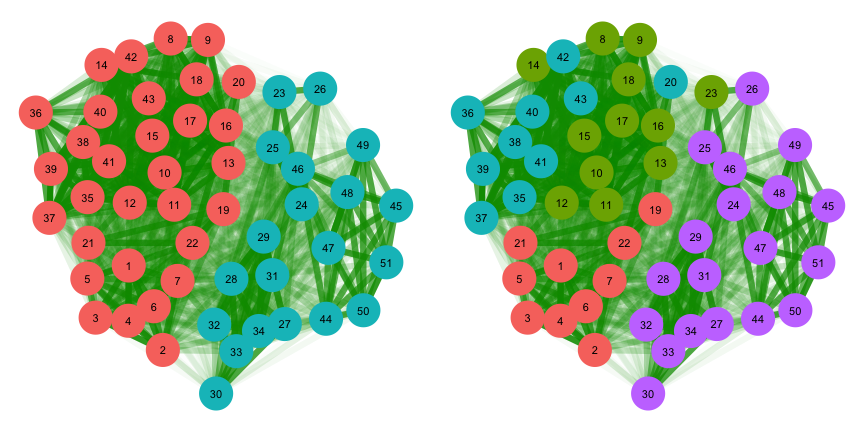## Automatically find the best number of clusters

To find the global optimum for the number of clusters $$k$$, we can next create clusterings for all sensible number of clusters (from 2 to the number of IS - 1). For each $$k$$ we calculate a quality score, which is show in the following plot as a function of the number of target clusters. The best number of clusters is indicated in red:

bestNrCluster <- MultIS::find_best_nr_cluster(
data = dat,
sim = similarityMatrix,
method_reconstruction = "kmedoids",
method_evaluation = "silhouette",
return_all = TRUE)
plotDf <- data.frame(
k = as.numeric(names(bestNrCluster)),
score = as.numeric(bestNrCluster)
)
ggplot2::ggplot(plotDf, ggplot2::aes(x = k, y = score, group = 1)) +
ggplot2::geom_line() +
ggplot2::geom_point(ggplot2::aes(col = (score == max(score)))) +
ggplot2::scale_color_manual(values = c("TRUE" = "#FF0000", "FALSE" = "#000000")) +
ggplot2::theme_bw() +
ggplot2::theme(legend.position = "none")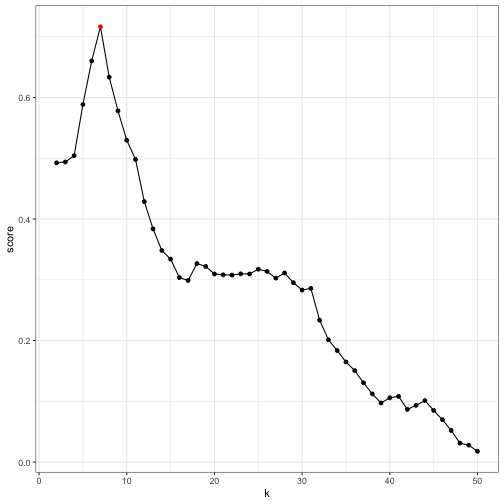The clustering for the optimal value of $$k$$ can either be obtained by selecting it from all evaluations of clusterings in the step beforehand, or we can just re-run the method and tell it to only give us the best number of clusters:

bestNrCluster <- MultIS::find_best_nr_cluster(
data = dat,
sim = similarityMatrix,
method_reconstruction = "kmedoids",
method_evaluation = "silhouette",
return_all = FALSE)


We then use this number $$k$$ to create a clustering and plot it. For the portrayed example, the data is best explained for $$k = 7$$ clusters:

clusterObjBest <- MultIS::reconstruct(
target_communities = bestNrCluster,
method = "kmedoids",
cluster_obj = TRUE,
sim = similarityMatrix)
plot(clusterObjBest)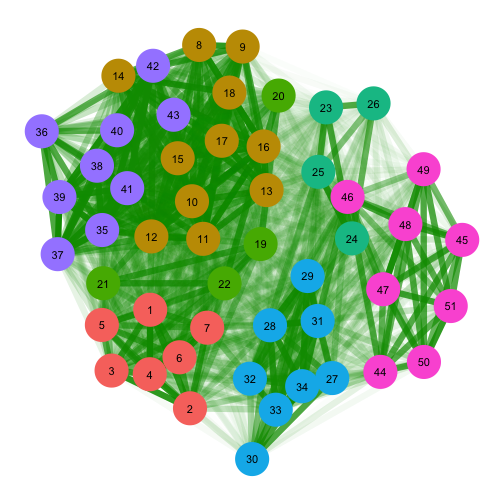# MultIS with known ground truth

For certain settings, such as model simulations or validation experiments, the true association between IS and clones is known. “MultIS” provides methods to integrate this information for benchmarking purposes.

Here, we use an illustrative example that was prepared with a simulation routine, which comprises the following steps:

1. Run a clonal simulation.
2. Add a multiplicative clonal variability to account for different cell types. This variability is beneficial to the reconstruction process.
3. Superimposed IS to the clones. The number of IS per clone is drawn from a Poisson distribution around a specified mean, estimating the average vector copy number (VCN).
4. Add Measurement noise multiplicatively to the IS counts to account for noise during the PCR and sequencing steps.

Each step in this analysis produces a time course that is the basis for the following step. In a real-world scenario, only the time course from the last step would be available, but as we use a simulation, we can use the known ground truth for validation and estimation of the accuracy of our methods.

First, the prepared example is loaded:

load("example.RData")


The loaded named list contains the steps of the simulation and further information. Its structure is the following:

str(simData, max.level = 1)
#> List of 7
#>  $params :List of 7 #>$ amplification_rates:List of 7
#>  $mapping : num [1:51, 1:2] 1 1 1 1 1 1 1 2 2 2 ... #> ..- attr(*, "dimnames")=List of 2 #>$ clone_counts       : 'matrix' num [1:7, 1:13] 150 150 150 150 150 150 150 463 526 492 ...
#>   ..- attr(*, "dimnames")=List of 2
#>  $clone_readouts : 'matrix' num [1:7, 1:13] 111.3 148.7 60.5 62.4 140.4 ... #> ..- attr(*, "dimnames")=List of 2 #>$ is_counts          : 'matrix' int [1:51, 1:13] 111 111 111 111 111 111 111 148 148 148 ...
#>   ..- attr(*, "dimnames")=List of 2
#>  $is_readouts : 'matrix' num [1:51, 1:13] 115.3 99.2 117.1 144.7 136.2 ... #> ..- attr(*, "dimnames")=List of 2  • params are the paramters, that were used to run the simulation, • ampRates are amplification rates that are applied to each IS, • mapping is a mapping from each clone to the contained ISs, • clone_counts is the raw result from the clonal simulation (step 1), • clone_readouts is the result after applying noise to the clonal simulation (step 2), • is_counts gives the raw IS readouts, i.e. after mapping ISs to the clones but before applying measurement noise (step 3), • is_readouts is the final result of our simulation routine (step 4). This adds measurement noise to the is_counts and would correspond to the data that is available in a real-world experiment. ## Time course representations The general structure for the time courses is again a table with the serial measurements at different time points in the columns and the different integration sites in the rows. The following is a small selection of simData$barcodeReadouts contained within the simData object:

Due to this data stemming from a simulation, we can look at all steps in the simulation, from the simulated clones to the integration sites:

p1 <- plot(simData$clone_counts) + ggplot2::ggtitle("Basic clonal simulation") p2 <- plot(simData$clone_readouts) + ggplot2:: ggtitle("Added clonal differences")
p3 <- plot(simData$is_counts) + ggplot2::ggtitle("Superimposition of integration sites") p4 <- plot(simData$is_readouts) + ggplot2::ggtitle("Added measurement noise")
gridExtra::grid.arrange(p1, p2, p3, p4, ncol = 2, nrow = 2)